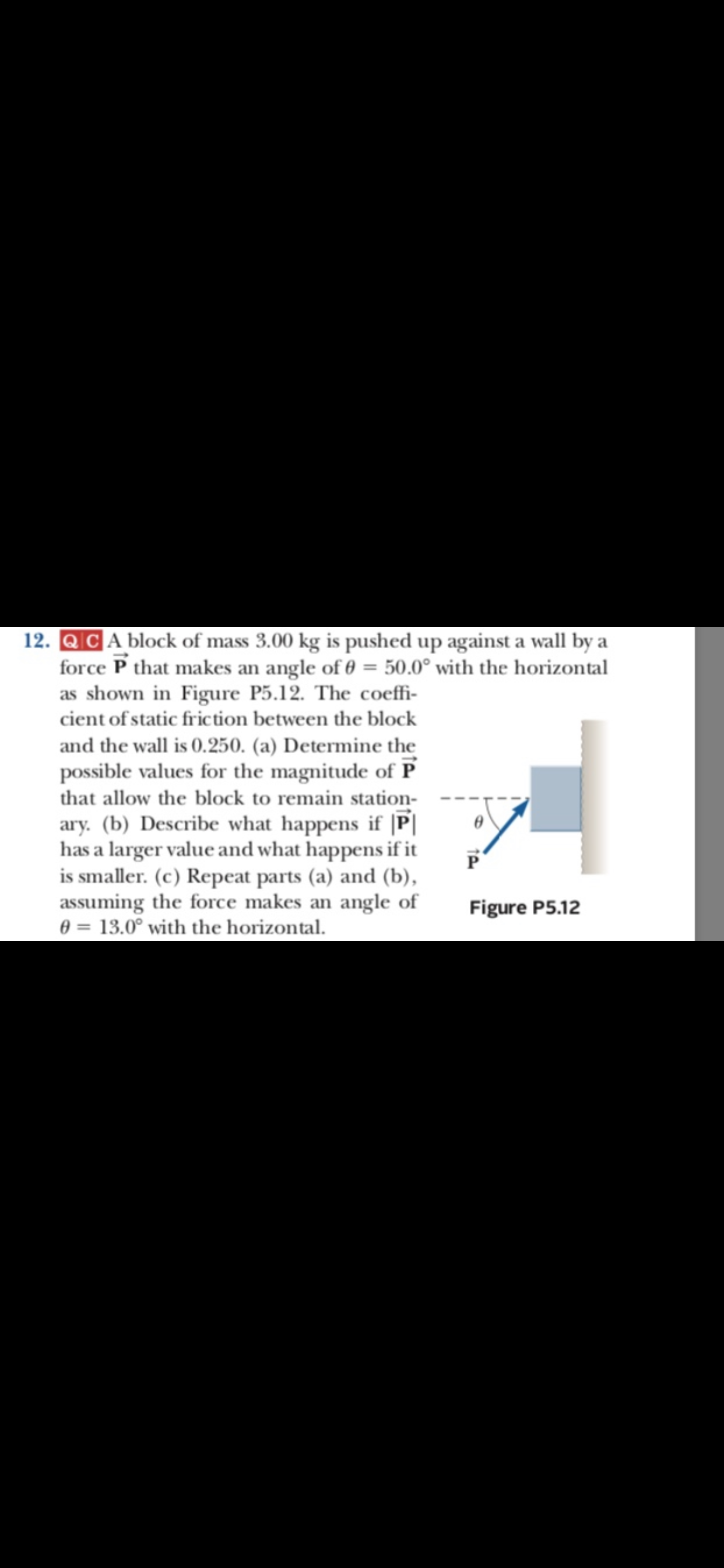# 12. QCA block of mass 3.00 kg is pushed up against a wall by a force P that makes an angle of 0 50.0° with the horizontal as shown in Figure P5.12. The coeffi- cient of static fric tion between the block and the wall is 0.250. (a) Determine the possible values for the magnitude of P that allow the block to remain station- ary. (b) Describe what happens if |P has a larger value and what happens if it is smaller. (c) Repeat parts (a) and (b), assuming the force makes an angle of 0 13.0° with the horizontal. Figure P5.12

Questionhelp_outlineImage Transcriptionclose12. QCA block of mass 3.00 kg is pushed up against a wall by a force P that makes an angle of 0 50.0° with the horizontal as shown in Figure P5.12. The coeffi- cient of static fric tion between the block and the wall is 0.250. (a) Determine the possible values for the magnitude of P that allow the block to remain station- ary. (b) Describe what happens if |P has a larger value and what happens if it is smaller. (c) Repeat parts (a) and (b), assuming the force makes an angle of 0 13.0° with the horizontal. Figure P5.12 fullscreen

### Want to see the step-by-step answer?